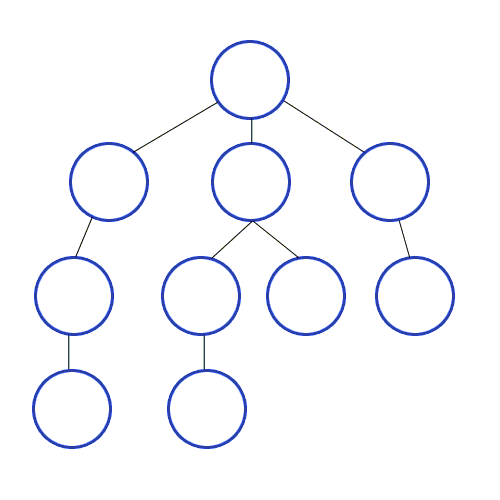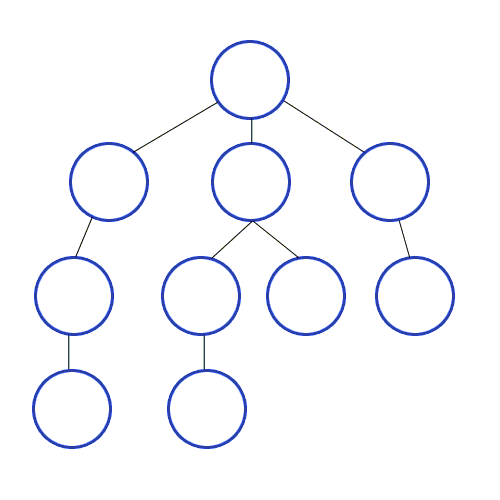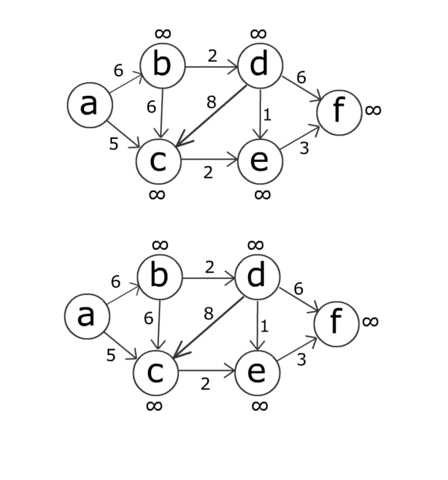Algorithm Runtimes

On studying for my final exam, I found that thinking about the algorithms in a cumulative manner made runtime memorization very simple.

BFS/DFS

At the simplest level, we have breadth-first search and depth-first search. These two algorithms are true to their name and cycle through the graph in O(V+E) time because they cover every edge and every vertex.

BFSDFSSingle Source Shortest Path

Bellman-Ford’s runs in O(VE) time goes through each vertex (O(V)) and “relaxes” all edges (O(E)).
Dijstra’s is (usually) an improvement on this and runs in O(E+Vlg(V)) time. However, you need non-negative weights, as this algorithm could get caught in an endless cycle.

Note: Bellman-Ford can be handy when the shortest path length is known, as you can bound E. In contrast, Dijstra’s would still run in O(E+Vlg(V)) time.All-Pairs Shortest Path

APSP builds on SSSP, but has to additionally look at the shortest path from EACH of the edges. So all the runtimes are multiplied by V.

Floyd-Warshall runs in worst case O(V^3). Johnson’s Algorithm takes advantage of Dijstra’s Algorithm, and runs it over each vertex. So, O(V)* O(E+Vlg(V)) = O(VE + V^2lg(V))

Flow Algorithms

Flows are simply graphs with a source and a sink.

Ford-Fulkerson goes over each edge a total of f times, since this is the amount of possible residuals.
To bound f, one can run a BFS/DFS to select an optimal residual path to get the Edmunds-Karp algorithm, which runs in O(VE^2)

Here is a data structure runtime summary

Here is an algorithm runtime summary